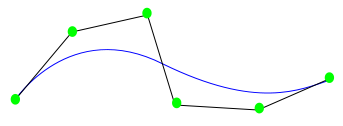# NURBS Curves

A NURBS curve is a parametric HOOPS primitive, i.e., it’s geometric properties are determined by a set of mathematical functions. For drawing purposes, however, a NURBS curve will inherit from the segment any attributes (visibility, color, line pattern) as if it were a polyline.

A detailed description of NURBS curves is beyond the scope of this document. We recommend “The NURBS Book” by Piegl and Tiller as a reference on the topic. That said, NURBS curves can be summarized as having the following properties

• Curve position is determined as a weighted average of its control points.

• The contribution of a given control point is determined by the parameter, “u” (varies from 0 to 1), the degree of the curve, the “knot vector” (see below), and the explicit weights that are provided by the user. Although negative control point weights are not part of the specification, they are handled correctly within HOOPS Visualize. Point weights cannot all be zero.

• High-degree NURBS curves, like high-degree polynomials, maintain continuity of high-order derivatives.

• The knot vector is simply a non-decreasing array of floats, whose values need not necessarily be unique.

The knot vector has a rather complex way of affecting control point contributions that is best left to a detailed reference on the subject, except to say the following:

• Repeated or closely spaced knot values will cause a curve to come near a particular control point.

• Repetition of a knot value for a number of times equal to the curve degree will cause the curve to touch a control point. This is often used at the start and end of the curve to make the curve touch its endpoints.

Most of the things that one can do with NURBS curves can also be done with polylines. The only thing that cannot be done by inserting a polyline instead is view-dependent tessellation. By using NURBS curves users can zoom in to a curve indefinitely without getting noticeable faceting artifacts.A NURBS curve in blue with its control points in green.

## NURBS Curves Example

The following snippet inserts a NURBS curve in the shape of a circle.

```    float pts = {{1, 0, 0}, {1, -1, 0}, {0, -1, 0}, {-1, -1, 0}, {-1, 0, 0}, {-1, 1, 0}, {0, 1, 0}, {1, 1, 0}, {1, 0, 0}};
float weights = {1, 0.707f, 1, 0.707f, 1, 0.707f, 1, 0.707f, 1};
float knots = {0, 0, 0, 1, 1, 2, 2, 3, 3, 4, 4, 4};

HC_Insert_NURBS_Curve(2, 9, pts, weights, knots, 0, 1);
```

## Precision Control

Refer to the curves section of the Programming Guide for details on how to control NURBS curve tesselation.

## Curve Trimming

Two of the arguments passed into ::Insert_NURBS_Curve are `start_u` and `end_u`. These define a simple 1-dimensional form of trimming for NURBS curves. This is particularly useful for importing IGES data, which has such parameters to define the start and end. To make the entire curve visible, values of 0.0 and 1.0 can be passed in for the curve `start_u` and `end_u` parameters. Alternatively, to make the first half of the curve visible, use 0.0 and 0.5.

Keep in mind that the curve parameterization always going from 0 to 1 does not match some solid modellers. Instead, solid modeling kernels tend to parameterize with respect to their knot vector. For example, such a modeling kernel would believe that a 3 point quadratic curve with a knot vector `{0.2, 0.3, 0.4, 0.5, 0.6, 0.7}` would span the range of 0.4 to 0.5. The `start_u` and `end_u` would then be expected to be in that range (e.g. `[0.41, 0.47]`). Use the following formula to convert from the solid modeller parameterization to what HOOPS expects:

`````start_u``_hoops = (``start_u``_modeler - knots[degree]) / (knots[control_point_count] - knots[degree]);
``end_u``_hoops = (knots[control_point_count] - ``end_u``_modeler) / (knots[control_point_count] - knots[degree]);
```

## Limitations

HOOPS Visualize does not support a knot multiplicity greater than the order (degree of the curve plus one), as this would cause the curve to be completely disjoint at that point. Additionally, since NURBS curves are only defined when knot vectors are non-decreasing, such curves are not supported by HOOPS. Point weights cannot all be zero.

It is not currently possible to set a “draw 3d nurbs curve” callback, though such functionality may come in the future if and when there is sufficient interest.